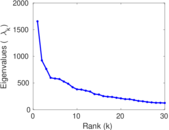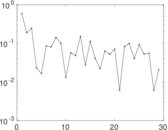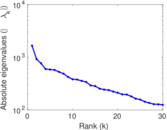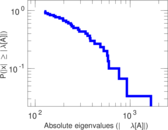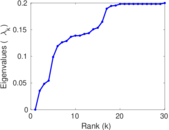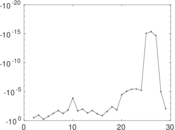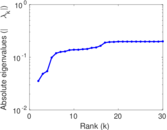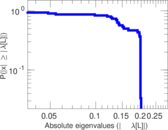# Wiktionary edits (hu)

This is the bipartite edit network of the Hungarian Wiktionary. It contains users and pages from the Hungarian Wiktionary, connected by edit events. Each edge represents an edit. The dataset includes the timestamp of each edit.

 Code `mhu` Internal name `edit-huwiktionary` Name Wiktionary edits (hu) Data source http://dumps.wikimedia.org/ AvailabilityDataset is available for download Consistency checkDataset passed all tests Category Authorship network Dataset timestamp 2017-10-20 Node meaning User, article Edge meaning Edit Network formatBipartite, undirected Edge typeUnweighted, multiple edges Temporal dataEdges are annotated with timestamps

## Statistics

 Size n = 388,013 Left size n1 = 1,424 Right size n2 = 386,589 Volume m = 2,150,545 Unique edge count m̿ = 1,345,399 Wedge count s = 52,198,980,769 Claw count z = 2,188,290,718,303,292 Square count q = 31,989,405,344 4-Tour count T4 = 464,713,924,542 Maximum degree dmax = 305,940 Maximum left degree d1max = 305,940 Maximum right degree d2max = 722 Average degree d = 11.084 9 Average left degree d1 = 1,510.21 Average right degree d2 = 5.562 87 Fill p = 0.002 443 95 Average edge multiplicity m̃ = 1.598 44 Size of LCC N = 384,396 Diameter δ = 12 50-Percentile effective diameter δ0.5 = 3.075 27 90-Percentile effective diameter δ0.9 = 3.847 03 Median distance δM = 4 Mean distance δm = 3.132 38 Gini coefficient G = 0.777 000 Balanced inequality ratio P = 0.197 322 Left balanced inequality ratio P1 = 0.028 150 5 Right balanced inequality ratio P2 = 0.284 112 Relative edge distribution entropy Her = 0.672 844 Power law exponent γ = 2.070 16 Tail power law exponent γt = 4.511 00 Tail power law exponent with p γ3 = 4.511 00 p-value p = 0.000 00 Left tail power law exponent with p γ3,1 = 1.401 00 Left p-value p1 = 0.000 00 Right tail power law exponent with p γ3,2 = 8.191 00 Right p-value p2 = 0.421 000 Degree assortativity ρ = −0.241 505 Degree assortativity p-value pρ = 0.000 00 Spectral norm α = 1,655.77 Spectral separation |λ1[A] / λ2[A]| = 1.794 05 Controllability C = 382,153 Relative controllability Cr = 0.992 891

## Plots

### Fruchterman–Reingold graph drawing### Degree distribution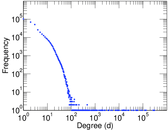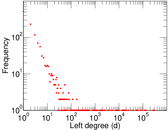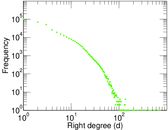### Cumulative degree distribution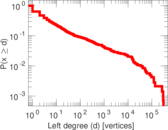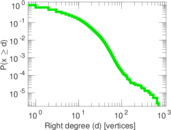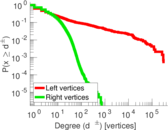### Lorenz curve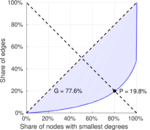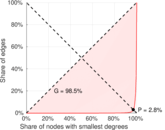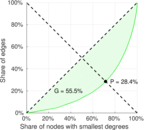### Spectral distribution of the adjacency matrix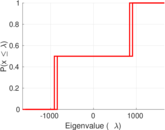### Spectral distribution of the normalized adjacency matrix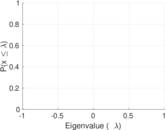### Spectral distribution of the Laplacian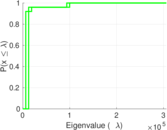### Spectral graph drawing based on the adjacency matrix### Spectral graph drawing based on the Laplacian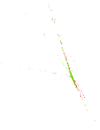### Spectral graph drawing based on the normalized adjacency matrix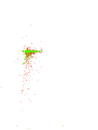### Degree assortativity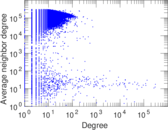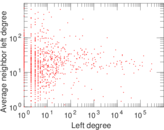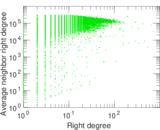### Zipf plot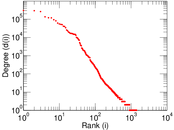### Hop distribution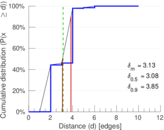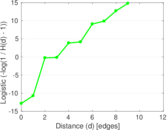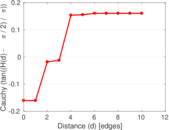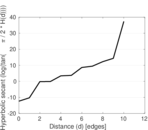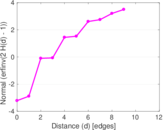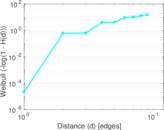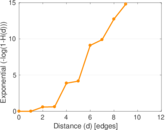### Delaunay graph drawing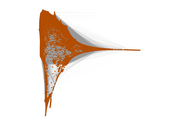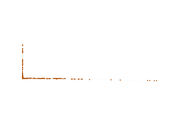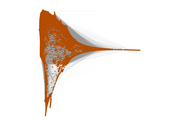### Edge weight/multiplicity distribution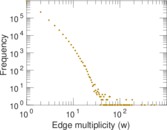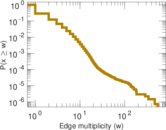### Temporal distribution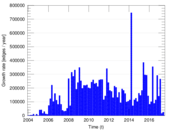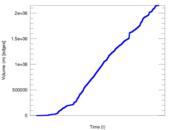### Diameter/density evolution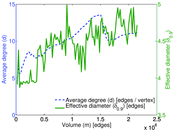### Matrix decompositions plots